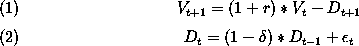### An example calling the MATLAB Implementation of AIM

This example uses a simple two-equation model to demonstrate how to use the MATLAB implementation of the Anderson-Moore Algorithm.

##### The Firm Value Model

We investigate the solution of a simple linear model which describes the value of a firm. We investigate the solution of a simple linear model which describes the value of a firm.

The model consists of two equations:where V is the value of the firm, D is the dividend, r is the interest rate,is the growth rate of the dividend (here, negative). E is the error term for stochastic shocks.

##### Using the Anderson Moore Algorithm

The AIM routine requires three input files:

1. A model file in syntax called MODELEZ
2. A parameter file.
3. The setup script to execute AIM.
##### Model Representation in MDLEZ

``````Here is what the MODELEZ  file for our example looks like.

Below we will explain each line of the file.```

MODEL> FIRMVALUE

ENDOG>
V
DIV
e_
one

EQUATION> VALUE

EQUATION> DIVIDEND
EQ>     DIV = (1-DELTA)*LAG(DIV,1)

EQUATION>  e_
EQ>        e_ = 0 * one

EQUATION>   ONE
EQ>         one = LAG(one,1)

END
```

MODEL>
The name of the model.

ENDOG>
The names of the endogenous variables. In the AIM formulation, models must completely describe the long-run behavior of the system. Thus all variables are endogenous. "Exogenous variables must have, at least, a trivial forecasting equation.

EQUATION>
The name of the equation.

EQ>
The model equation definition.

`The parameter file for our example is called firmparms.m: `

```
R = 0.10;
DELTA = 0.60;
```

`The following script, which we call runfirm.m, executes AIM for the firmvalue model: `

```
dirnam='./'; % directory of this example
parnam='firmparms';
modnam='firmvalue';
[cof, scof, cofb, param_, eqname_,...
endog_, eqtype_, vtype_, neq, nlag, nlead, rts, lgrts,aimcode]=...
SPSolve(dirnam, modnam, parnam);
```

`Sample run of the firmvalue model on Unix: `

Change the directory to the directory where the script resides:
The script to run aim is: runfirm.m
The model file name is: firmvalue
The parameter file name is: firmparms.m

```

mqmx3(174)% cd ~/yourPathTo/examples/firm
```

Call matlab and execute the run script

```
msulx1(124)% matlab -nodesktop

< M A T L A B >
Version 7.0.4.352 (R14) Service Pack 2
January 29, 2005

To get started, type one of these: helpwin, helpdesk, or demo.
For product information, visit www.mathworks.com.

>> runfirm
runfirm
SPSolve version:(\$Name:  \$,\$Revision: 1.4 \$)

ans =

found Aim on system classpath

initializing parsers
Aim Java Parser Version 1.03: Reading from file ./firmvalue...
Aim Java Parser Version 1.03: No syntactic errors found.
Checking for errors......
No semantic errors found.
chose modelez parser
>>
```

Displaying the results:

In the output below:
"cof" is the structural coefficients matrix (neqs by neqs*(nlag+nlead+1))
"cofb" is the reduced form coefficients matrix (neqs by neqs * nlag)
"scof" is the observable structure matrix (neqs by neqs*(nlag+1))
"rts" returns the eigenvector
"aimerr(aimcode)" returns a diagnostic message about the model

```where: "neq" = number of equations
"nlag" =number of lags
```

```
> cof
cof

cof =

Columns 1 through 7

0         0         0         0   -1.1000         0         0
0   -0.4000         0         0         0    1.0000   -1.0000
0         0         0         0         0         0    1.0000
0         0         0   -1.0000         0         0         0

Columns 8 through 12

0    1.0000    1.0000         0         0
0         0         0         0         0
0         0         0         0         0
1.0000         0         0         0         0

> cofb
cofb

cofb =

0    0.2286         0         0
0    0.4000         0         0
0         0         0         0
0         0         0    1.0000

> scof
scof

scof =

Columns 1 through 7

0         0         0         0   -1.1000    0.6286         0
0   -0.4000         0         0         0    1.0000   -1.0000
0         0         0         0         0         0    1.0000
0         0         0   -1.0000         0         0         0

Column 8

0
0
0
1.0000

> rts
rts

rts =

1.1000
1.0000
0.4000

> aimerr(aimcode)
aimerr(aimcode)

ans =

Aim: unique solution.
```
##### References

1 Gary Anderson. A reliable and computationally efficient algorithm for imposing the saddle point property in dynamic models. Unpublished Manuscript, Board of Governors of the Federal Reserve System, 1997.

2 Gary Anderson and George Moore. A linear algebraic procedure for solving linear perfect foresight models. Economics Letters, 17, 1985.

3 Michael W. Berry. Large scale sparse singular value computations. University of Tennessee, Department of Computer Science, 1996.

4 Olivier Jean Blanchard and C. Kahn. The solution of linear difference models under rational expectations. Econometrica, 48, 1980.

5 Gene H. Golub and Charles F. van Loan. Matrix Computations. Johns Hopkins, 1989.

6 E. V. Krishnamurthy. Parallel Processing: Principles and Practice. Addison-Wesley, 1989.

7 David G. Luenberger. Time-invariant descriptor systems. Automatica, 14:473-480, 1978.

8 Ben Noble. Applied Linear Algebra. Prentice-Hall, Inc., 1969.

9 J. Taylor. Conditions for unique solutions in stochastic macroeconomic models with rational expectations. Econometrica, 45:1377-1385, |SEP| 77.

10 C. H. Whiteman. Linear Rational Expectations Models: A User's Guide. University of Minnesota, 1983.